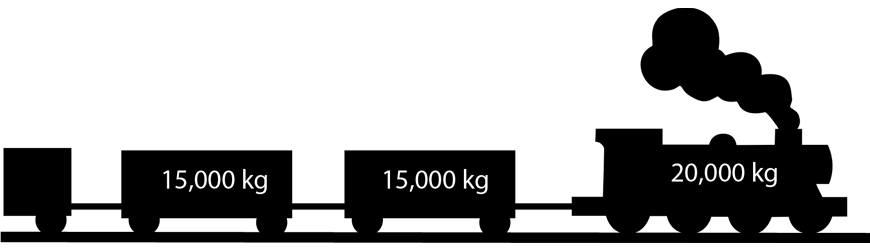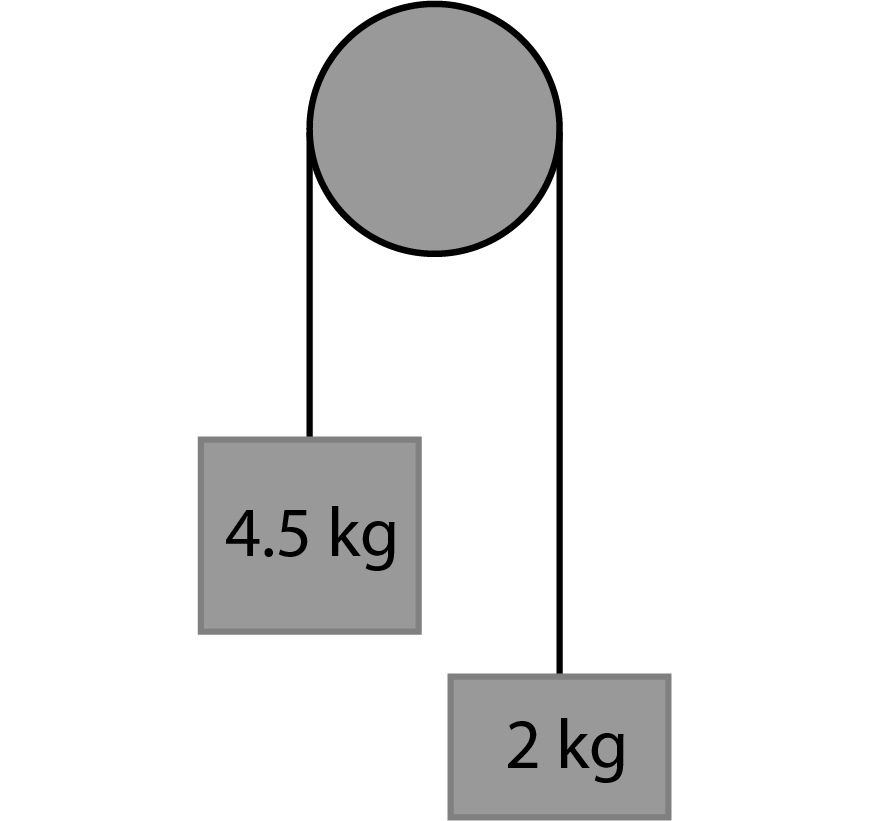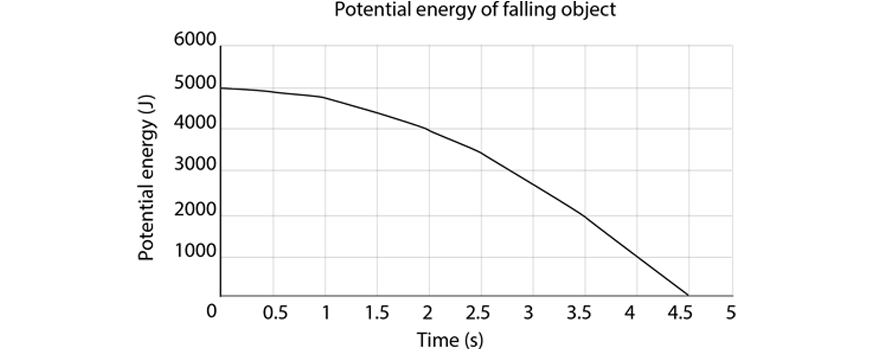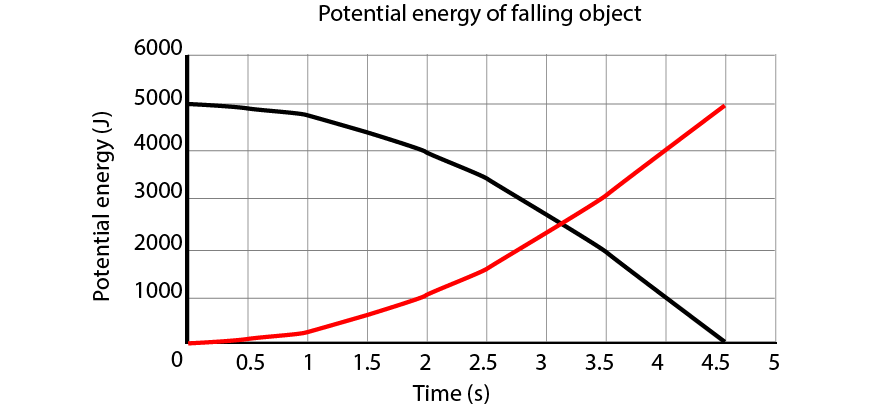# 2019 Year 11 Physics: Dynamics Practice Questions

Are your yearly exams around the corner? Well, we've put together ten questions for you to test your skills on!## 2019 Year 11 Physics: Dynamics Practice Questions

Are you ready for your Yearly exams? In this post, we’ve shared ten challenging Physics: Dynamics questions. Test your knowledge and skills and then check your responses with these solutions at the bottom of the page.

## Dynamics Questions

### Question 1 (5 Marks)

A $$10\text{ kg}$$ block is placed on a slope inclined at an unknown angle, as shown below. The surface is not frictionless.a) Indicate the forces acting on the block in the above diagram.

b) If the coefficient of static friction is $$\mu_s = 0.45$$, show that the maximum slope angle before the block starts to slide is $$24.2^{\circ}$$.

c) The coefficient of kinetic friction is $$\mu_k = 0.3$$. Calculate the net force on the block once it begins sliding, if the angle of the slope is $$24.2^{\circ}$$.

d) Calculate the distance along the slope the box slides after $$2\text{ s}$$.

### Question 2 (3 Marks)

A $$5\text{ kg}$$ block is being pulled from rest along a flat surface by an oblique force, as shown in the diagram below. The coefficient of static friction is $$\mu_s = 0.34$$, and the coefficient of kinetic friction is $$\mu_k = 0.3$$.a) Determine if the block will slide or remain stationary.

b) Calculate the work done by kinetic friction on the block if it slides over a distance of $$5\text{ m}$$.

### Question 3 (3 Marks)

A series of carriages are being pulled along a frictionless track by an engine car. The engine car can exert a force of $$200,000\text{ N}$$ and has a mass of $$20,000\text{ kg}$$a) Calculate the maximum acceleration of the engine car, if there are no carriages attached.

b) Each carriage has a mass of $$15,000\text{ kg}$$. Calculate the maximum number of carriages the engine can pull with an acceleration of at least $$0.5 \text{ ms}^{-2}$$.

c) If the engine pulls 10 carriages, determine the maximum tension force within any of the linkages.

### Question 4 (3 Marks)

Two masses are hanging from a frictionless pulley as shown below:a) Calculate the net force acting on the two masses.

b) Determine the resultant acceleration of the system.

c) Calculate the tension in the string between the two blocks.

### Question 5 (3 Marks)

An object resting on a table experiences two forces acting on it in the configuration below:a) Calculate the resultant force on the object.

b) A third force is applied to cancel the effects of the first two, allowing the object to remain in equilibrium. Calculate the magnitude and direction of this force.

### Question 6 (4 Marks)

A $$50\text{ kg}$$ object undertakes a roller-coaster motion as shown below. The object begins at rest at position A.a) What is the total mechanical energy of the object at position A?

b) What is the speed of the object at position B?

c) At position C, the object has slowed to $$10\text{ ms}^{-1}$$. What is the height of position C above position B?

d) After the object descends the final slope, a brake pad brings the object to rest via a constant braking force of $$1600 \text{ N}$$. What is the distance the object travels along this brake pad before coming to rest?

### Question 7 (3 Marks)

A warehouse worker is lifting a series of boxes onto higher shelves for storage.

a) Determine which of the following actions requires more work:

• Lifting a $$10\text{ kg}$$ box up $$1.5 \text{ m}$$
• Lifting a $$30 \text{ kg}$$ box by $$0.25 \text{ m}$$

b) In one hour, the worker lifts a $$10\text{ kg}$$ box a cumulative total of $$350 \text{ m}$$. Determine their average power output.

c) The worker attempts to push a heavy box weighing $$50\text{ kg}$$ to the other side of the warehouse. Calculate the maximum speed the worker can push the box, if the coefficient of kinetic friction between the box and floor is $$\mu_k=0.10$$ and the worker’s power output is that determined in b).

### Question 8 (4 Marks)

A $$13 \text{ kg}$$ object is dropped from rest from a certain height. The gravitational potential energy over time for the object (ignoring air resistance) is shown below:a) On the same axes, graph the kinetic energy of the object.

b) Use the graph to estimate the starting height of the object.

c) Calculate the object’s downward velocity at time $$t=3.5\text{ s}$$.

### Question 9 (4 Marks)

A tennis ball with a mass of 50 g is thrown directly at a brick wall. The ball rebounds in the exact opposite direction at the same speed. A plot of the force over time on the tennis ball is shown below:a) Determine the average acceleration of the ball during the collision with the wall.

b) Calculate the total change in momentum of the ball.

c) Outline the significance of the area under the graph.

d) Calculate the initial speed of the ball.

### Question 10 (4 Marks)

A car ($$m=2,000\text{ kg}$$, $$u=10\text{ ms}^{-1}$$) and a truck ($$M=10,000\text{ kg}$$, $$u=7.45\text{ ms}^{-1}$$) are involved in a head-on collision. After the collision, the two bodies move together as one

a) State weather the collision is elastic or inelastic, giving reasons.

b) Calculate the combined momentum of the car and truck before the collision.

c) Determine the velocity of the car and truck after the collision.

d) State which of the two objects (car or truck) experiences a larger force, and why.

## Need more practice with Dynamics?

Get ahead of your peers with Year 11 Holiday Accelerated Courses. With advanced completion of contents before it’s taught at school, you’ll be better prepared for your school assessments.

Book your spot in our Year 11 Accelerated Physics today and join over 4500 students who already have a head start## Solutions

Question 1:

a)b) The maximum angle of the slope for which the friction balances the weight can be expressed as $$tan \theta = \mu$$. Hence: $$\theta = tan^{-1}(\mu_{s}) = tan^{-1}(0.45) = 24.2^\circ$$

c) $$13.4 \text{ N}$$ down the slope

d) $$2.67 \text{ m}$$

Question 2:

a) Force from static friction is $$12.94\text{ N}$$, the horizontal component of the applied force is $$30.07\text{ N}$$. Therefore the object will slide. Note that the normal force is reduced due to the upwards component of the applied force.

b) $$-57.1\text{ J}$$

Question 3:

a) $$10 \text{ ms}^{-2}$$

b) $$25 \text{ carriages}$$

c) $$176,471 \text{ N}$$

Question 4:

a) $$24.5 \text{ N}$$, downward for the larger mass.

b) $$3.77 \text{ ms}^{-2}$$, downward for the larger mass.

c) $$27.14 \text{ N}$$

Question 5:

a) $$271 \text{ N, S86}^{\circ}\text{E, or }94^{\circ}\text{T}$$

b) $$271 \text{ N, N86}^{\circ}\text{W, or }274^{\circ}\text{T}$$

Question 6:

a) $$14,700 \text{ J}$$

b) $$24.3 \text{ ms}^{-1}$$

c) $$24.9 \text{ m}$$

d) $$9.19 \text{ m}$$

Question 7:

a) Lift 1: $$147 \text{ J}$$
Lift 2: $$73.5\text{ J}$$
The first lift requires more work done.

b) $$9.53 \text{ W}$$

c) $$0.2 \text{ ms}^{-1}$$

Question 8:

a)b) Approximately $$39 \text{ m}$$

c) Approximately $$21.5 \text{ ms}^{-1}$$

Question 9:

a) $$400\text{ ms}^{-2}$$ away from the wall

b) $$0.6\text{ kgms}^{-1}$$ away from the wall

c) The area under a force time graph is the impulse, which is also the change in momentum.

d) $$6\text{ ms}^{-1}$$

Question 10:

a) Collision is inelastic, as the two bodies move together as one after the collision – this only happens for inelastic collisions.

b) $$54,500\text{ kgms}^{-1}$$ in the direction of the truck’s initial velocity.

c) $$4.54 \text{ ms}^{-1}$$ in the direction of the truck’s initial velocity.

d) The forces experienced by each are the same (Newton’s Third Law)